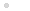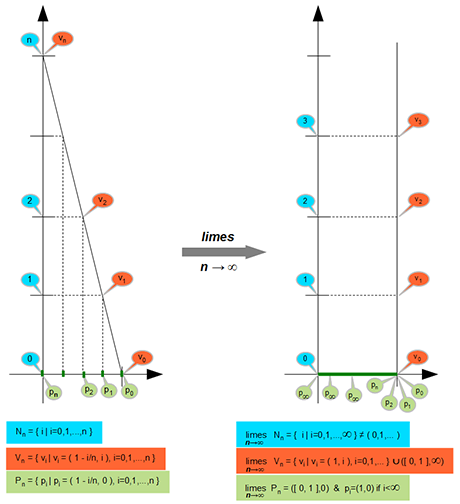### Leírás

(0,1,2,3,..) ≠ {0,1,2,3,...}
______________________
Ezek a gondolatok át fogják formálni a matematikát. De hogy miről szól a blog, azt az áttekintőben olvashatod. 2012 júliusa óta nem tudott senki cáfolatot adni. Ez már-már az igazolásom.
______________________
These ideas will be to shape the mathematics. But what I'm talking about the blog, you can read about it in the overview. Since July 2012 could no rebuttal. This is almost proof.

### 2014.10.13. 16:55 | Takács Ferenc bp. | Szólj hozzá!

Here are a simple mappings of point sets. The first point set is the starting slices sets of set of natural numbers (N) and the second point set is integer coordinates of the straight relying on against vertical axis (V) and the third point set is projection of V set to the horizontal axis (P). The straight line intersects the horizontal axis at value 1, and intersects the vertical axis is a positive integer n coordinate, and n is the index of the point set. According to a indices of sets of indices series are formed, and then and we take the limits of this series.Thus on the first image visible two mapping (N => V and V => P) and on the second image visible these limits.

The series of limit training it turns out that the index set, ie the set of finite natural numbers is not map to limits set, because the limits set of set of point projection is the continuous interval of the real numbers, and the limit of ladder points is vertical series of points over coordinate one, and projections of all finite index of these points series  is coordinate one. So voluntarily leads to the solution to the remainder of the interval, which is the complete intervale except for one point, is based on the infinite values of indices. With the dissolves the contradiction is that the countably infinite finite index it can not one-to-one map to an uncountable infinite continuous interval. Indeed, it does not maps, because all finite indices map to a single point and only the infinite indices can map to continuous interval, which is therefore uncountable endless.

This also means that the series of the finite natural numbers (which defined with the operation subsequent)  just countably infinite, and the set of all natural numbers, which obtained by the limit of this series, is uncountable infinite and contains the infinite numbers. Therefore, references to the set of natural numbers imply a reference to the infinite large numbers, which is usually not desirable, because with them can not be carried out all the operations that can be specified with the finite numbers. Also problem reference to the set of rational numbers, since the presence of the infinite numbers this set of is the same as the set of real numbers. So the rational numbers can only sensibly be defined as a series rather than as a set.

To better understand the difference between the concepts of the infinite set and  the series, just think of the principle of mathematical induction. This principle gives the key to prove the theoretical problems which formulated in the finite natural numbers. The principle of mathematical induction in perfect harmony with defining the concept of the series which of course is not surprising given the natural numbers form a series, and the principle of mathematical induction requires us to have have a proven proposition also create an infinite series of assertion such members per order is equivalent to the set of natural numbers, so that claim is valid for all natural numbers. This requirement is necessary and sufficient condition for to prove the truth of assertion. This also means that any additional requirement unnecessary and wrong regarding to statements among the natural numbers, I am thinking primarily the treatment the series of natural numbers as a set. As can be seen above from the example the endless ladder that in the V limit set can not be distinguished definitely finite and infinite indices members. Although can to say that x coordinate of every finite index tag  equal one, but do not to say that every tags with one x coordinate is finite index. So all finite natural numbers is not separated definitely from the infinite numbers, so we not can count with elements of such a set if they would only finite natural numbers.

This is closely related to the fact that in the case of series and infinite sets the power set (or those series) have the same cardinality as the base sequence or set, therefore the Georg Cantor's theorem, which states the contrary, is wrong.

Budapest, 2013. április 26.

### A bejegyzés trackback címe: